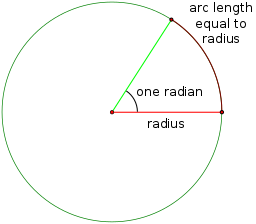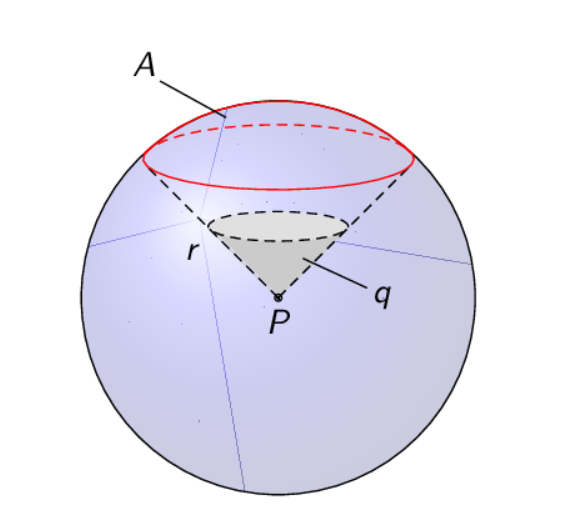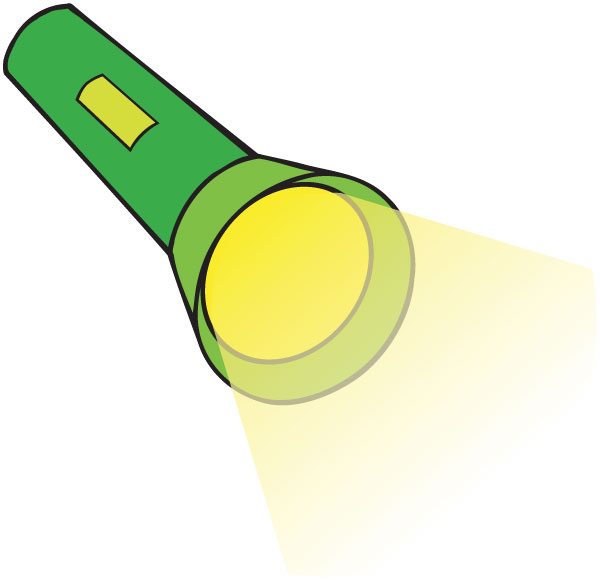# Year 10+ 3D Geometry

### Chapters

Physicists use a unit called a steradian to measure "solid" angles when they encounter problems in 3-dimensional geometry. Steradians are particularly important in astronomy and in optics, but they arise in any field where physicists need to study the flow of particles through a given area.

You might notice that the term "steradian" looks a bit like "radian". Steradians are related to spheres in much the same way as radians are related to circles:A radian is subtended by an arc on the circle's circumference that has the same length as the radius of the circle.

A steradian is subtended by an area on the sphere's circumference equal to the radius of the sphere squared.Another term for a steradian is a square radian. The abbreviation for steradian is sr.

## How many steradians in a sphere?

As the surface area of a sphere is given by the formula $S = 4 \pi r^2$, where $r$ is the radius of the sphere, and the area subtended by a steradian is equal to $r^2$ square units, the sphere contains $\dfrac{4\pi r^2}{r^2} = 4 \pi$ steradians. Recall that the circle contains $2\pi$ radians.

This means that the area carved out by a steradian is equal to $\dfrac{1}{4\pi} \approx 0.08$ parts of a sphere. Note that this doesn't depend on the radius of the sphere, just as the number of radians in a circle doesn't depend on the radius of the circle. We are measuring angles, not lengths.

## Is there an equivalent solid angle measure to degrees?

Yes, there is. It's called square degrees. You can convert from steradians to square degrees in much the same way as you convert from radians to degrees:

• $1$ radian is equal to $\dfrac{180}{\pi}$ degrees.
• $1$ steradian is equal to $\left(\dfrac{180}{\pi}\right)^2$ square degrees.

Note that we square the radian to degrees conversion for steradians to degrees, just as we square the radius when we are defining the steradian.

## Luminous IntensityThe steradian pops up in the units for luminous intensity (lumens per steradian). A lumen is the quantity of light that flows from a given light source in any second. The luminous intensity of a light source is the amount of visible light that it emits per unit time, per unit of solid angle. These measurements are very important in astronomy and optics. You might actually see a luminous intensity rating if you buy a specialist torch. Sam needs a high luminous intensity on the head torch he uses for caving.

### Description

There are several lessons related to 3D geometry such as

1. Euler's formula
2. Vertices, Edges and Faces
3. Volumes of 3D shapes
4. etc

Even though we've titled this lesson series to be more inclined for Year 10 or higher students, however, these lessons can be read and utilized by lower grades students.

### Prerequisites

Understanding of 3D shapes

### Audience

Year 10 or higher, but suitable for Year 8+ students

### Learning Objectives

Get to know 3D Geometry

Author: Subject Coach
You must be logged in as Student to ask a Question.